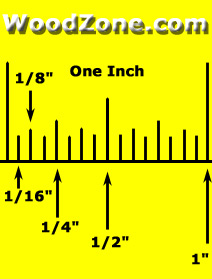# I Need To Learn How To Read A Ruler For A Test I Have To Take For A Job?

A ruler is extremely simple to read. Most rulers are 15 centimetres long but some are 30 centimetres. Along one side there are larger lines with a number next to them and this number indicates the length in the centimetres. In between the larger lines there are 9 little lines which indicate the millimetres. There are 10 millimetres in a centimetre. If you count five little lines after the 5cm mark, this would be five centimeters and five millimteters which is written 5.5cm. Nine little lines after the five would be 5.9cm. If you turn the ruler upside down you can also use it to measure inches. Inches are part of the old imperial system of measurement but some people do still use it so you may need to know how to measure in inches. The imperial system has not been on the school curriculum for many years in Britain, it is still extremely common to use feet and inches to measure height. Inches are longer than centimetres as there are 2.54cm in one inch. If you are measuring a line, hold the ruler against the line with the beginning of the ruler at the beginning of the line. The beginning of the ruler is the end which starts with ‘1’. Check where the line ends against the ruler and this is the length of the line. If you are measuring in centimetres and the line ends on the third little line after the five, then the line is 5.3cm. If you want to see how long it is in inches, simply turn the ruler upside down and the line will reach 2.08 inches.
thanked the writer.
A  ruler is divided into inches, centimeters and millimeters.
1 inch = 2.54 centimeter = 25.4 mm

There are 10 mm in one cm. Refer to my uploaded picture for clear understanding of ruler.thanked the writer.